# Introduction to Cryptography

Tom Kelliher, CS 325

Sept. 14, 2011

# Administrivia

### Assignment

Same reading.

Choose presentation topics. Grading criteria.

Perl/CGI lab.

### Outline

1. Basic terminology.

2. Substitution.

3. Transposition.

4. What makes a good cipher?

### Coming Up

More Cryptography.

# Basic Terminology

1. Plain text, cipher text.

2. Key.

3. Interceptor aims: block, intercept, modify, fabricate.

4. Symmetric cipher: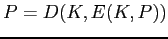.

One key. Key distribution and management issues. Private key cryptography.

5. Asymmetric cipher: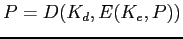.

Two keys: private and public. Public key cryptography.

6. Product Ciphers -- application of two or more ciphers: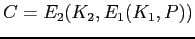.

The result is not necessarily ``better.''

7. Diffusion: A plain text character has a functional impact on multiple cipher text characters.

This forces the cryptanalyst to have access to large amounts of cipher text.

8. Confusion: A cryptanalyst should not be able to predict the changes that occur when one character of the plain text is changed. (Consider a Caesar cipher.)

This property makes it harder to understand the relationship between the plain text and the cipher text.

9. Stream cipher: One plain text character is used to produce one cipher text character.

Fast; no latency.

Poor diffusion; possibly little confusion.

10. Block cipher: A block of plain text is used to produce a block of cipher text.

Slower; latency.

Excellent diffusion and confusion.

# Substitution

1. Exchange one character for another, using a table. Many variations.

2. Simple examples: Caesar rotation, ROT13.

3. Permutation and keys.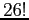possible permutations.

Simple permutation scheme using ``tolerant'' as key: toleranbcdfgh...
(Use key and follow up with remaining letters.)

4. One time pad. Components:
1. A set of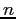permutations.

2. An infinite string of random numbers, modulo.

For each plaintext character, use the next random number to select the permutation to apply.

Keeping the pads in synch.

5. Other methods: Vernam cipher, book cipher.

# Transposition

1. Columnar technique.

2. Re-arrange the plaintext characters.

3. Primitive example: Pig Latin.

4. General idea:
1. Construct a matrix withcolumns.

2. Length of plain text should be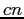. If not, pad out.

3. Write plain text across rows of matrix.

4. Read cipher text across columns of matrix.

# What Makes a Good Cipher?

1. According to Shannon:
1. Amount of secrecy should be proportional to amount of labor.

2. Keys, algorithms should be free from complexity.

3. Implementation process should be KISS.

4. Error in encrypting should not propagate.

5. Length of cipher text should match length of plain text.

2. ``Trustworthy'' encryption:
1. Based on sound mathematics.

2. Analyzed by experts and found to be sound.

3. Has withstood the ``test of time.''

Thomas P. Kelliher 2011-09-13
Tom Kelliher